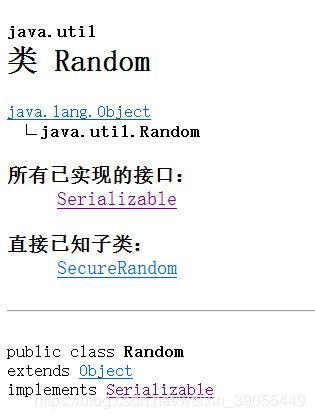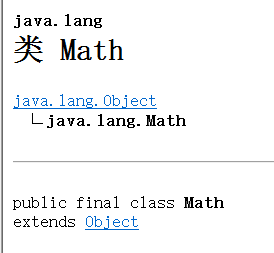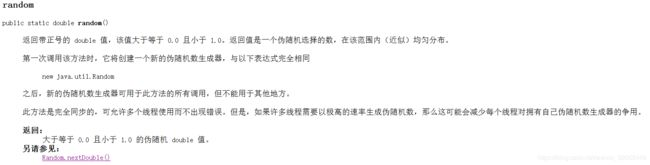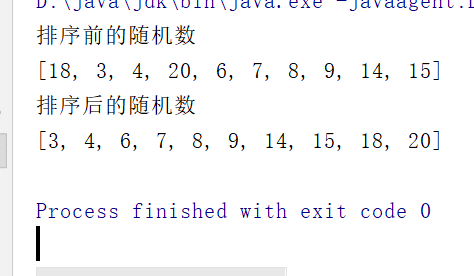我试图为我在1到60之间选择的每一行生成一个随机整数作为计时器。SELECT downloads.date, products.*, (FLOOR(1 + RAND() * 60)) AS timer我已经搜索并继续使用此FLOOR函数作为如何在范围内选择随机整数。这给我每一行1。我想念什么？我在mysql 5.0.75上我相信这是查询的其余部分，这可能是一个嵌套问题SELECT *FROM (SELECT downloads.date, products.*, FLOOR(1 + (RAND() * 60)) AS randomtimer,(SELECT COUNT( * )FROM distrosWHERE distros.product_id = products.product_id) AS distro_count,(SELECT COUNT(*) FROM downloads WHERE downloads.product_id = products.product_id) AS true_downloadsFROM downloadsINNER JOIN products ON downloads.product_id = downloads.product_id) AS count_tableWHERE count_table.distro_count > 0AND count_table.active = 1ORDER BY count_table.randomtimer , count_table.date DESC LIMIT 10
展开全文• 所以，拿到m-n之间的数，m不等于n，Math.round(Math.random()*(n-m)+m) 获取不重复的随机整数：（参数：随机数个数，最小值，最大值） function randomArr(params) { params = { ...{ length: 5, min: 2, max: 32 }...
Math.random()方法会返回介于 0（包含） ~ 1（不包含） 之间的一个随机数

假如想要拿到0-10之间的数，只需要将该方法的值*10 即Math.random()*10；

假如想要拿到0-20之间的数，同理，只需要将该方法的值*20  即Math.random()*20；

那么，想要拿到0-n之间的数，n是整十倍的数，即Math.random()*n。

想要拿到1-11之间的数呢？就是在0-10的基础上，加上1  即Math.random()*10+1；

即 想要拿到m-n+m之间的数，n是整十倍的数，即Math.random()*n+m。

拿到1-10之间的数呢？就是在0-9的基础上，加上1  即Math.random()*9+1；

即 想要拿到m-n之间的数，即Math.random()*(n-m)+m

此时，拿到的数值都是小数，而且都是大于m小于n的值。

舍掉小数点后的数值的方法有很多，如parseInt()，Math.ceil()，Math.floor()，Math.round()

如果使用parseInt()或Math.floor()，如在0-1之间，永远拿不到1的情况，不满足

如果使用Math.ceil()，如在0-1之间，除非拿到的值是0，否则都是拿到1的情况，虽然满足，但是概率不平均

使用Math.round()是最合适的，如在0-1之间，0.5及以上的值会返回1，0.5以下的值返回0。概率平均。

所以，拿到m-n之间的数，m不等于n，Math.round(Math.random()*(n-m)+m)

获取不重复的随机整数：（参数：随机数个数，最小值，最大值）

function randomArr(params) {
params = { ...{ length: 5, min: 2, max: 32 }, ...params };
let { length, min, max } = params;
if (
typeof length != "number" ||
typeof min != "number" ||
typeof max != "number" ||
max <= min ||
length <= 0
) {
return [];
}
if(max - min < length) {
length = max - min + 1;
}
let arr = [];
for (let i = 0; i < length; i++) {
let num = Math.round(Math.random() * (max - min)) + min;
if (!arr.includes(num)) {
arr.push(num);
} else {
i-=1
}
}
return arr;
}
var result0 = randomArr();
console.log(result0); // [ 7, 15, 18, 16, 4 ]
var result1 = randomArr({});
console.log(result1); // [ 17, 24, 27, 25, 8 ]
var result2 = randomArr({ length: 3 });
console.log(result2); // [ 24, 4, 27 ]
var result3 = randomArr({ min: 0 });
console.log(result3); // [ 21, 11, 8, 18, 13 ]
var result4 = randomArr({ max: 20 });
console.log(result4); // [3, 7, 11, 15, 8]
var result5 = randomArr({ max: 4 });
console.log(result5); // [3, 4, 2]


展开全文• 方式一：使用 Random 类举例：获取 0~3 范围内(包括 0 和 3 )的 int 类型的随机数Random random = new Random();System.out.println(random.nextInt(4)); //注意：这里的 4 指 0 1 2 3 四个数方式二：使用 Math 类的...
方式一：使用 Random 类举例：获取 0~3 范围内(包括 0 和 3 )的 int 类型的随机数Random random = new Random();System.out.println(random.nextInt(4)); //注意：这里的 4 指 0 1 2 3 四个数方式二：使用 Math 类的 random 方法举例：/*** 从键盘输入一个范围 [start,end], 获取该范围内的随机数。 注：[1, 5) 表示“左开右闭”，即： 1~5 包含 1，不包含 5*(int)(Math.random() * (end - start + 1) + start);* @author Rsbry*/public class GetRandomNumber {public static void main(String[] args){Scanner input = new Scanner(System.in);System.out.println("请输入取数范围(回车确认)");System.out.print("首：");int start = input.nextInt();System.out.print("尾：");int end= input.nextInt();System.out.print("十个随机数：");for(int i = 0; i < 10; i++){System.out.print(getRandom(start, end) + "\t");  //输出十个随机整数}}public static int getRandom(int start, int end){return (int)(Math.random() * (end-start+1) + start);}}输出结果：请输入取数范围(回车确认)首：52尾：100十个随机数：75648858756071545987
展开全文• 编写程序，获取10个1~~20的随机数，并进行排序 分析 使用 HashSet 容器来进行存储随机数 使用 TreeSet 来进行排序 程序代码 package com.company.test; import java.util.HashSet; import java.util.Random; import...
题目
编写程序，获取10个1~~20的随机数，并进行排序
分析
使用 HashSet 容器来进行存储随机数
使用 TreeSet 来进行排序
程序代码
package com.company.test;

import java.util.HashSet;
import java.util.Random;
import java.util.TreeSet;

public class MyTest {
public static void main(String[] args) {
//定义HashSer容器存储排序前且无重复的随机整数
HashSet<Integer> set1 = new HashSet<>();
while (set1.size() < 10) {
Random random = new Random();
//获取1--20的随机数
int num = random.nextInt(20) + 1;
}
System.out.println("排序前的随机数");
System.out.println(set1);
//定义TreeSet来对集合进行排序
TreeSet<Integer> set2 = new TreeSet<>(set1);
System.out.println("排序后的随机数");
System.out.println(set2);
}
}


运行结果展开全文random java
• 我试图为我在1到60之间选择的每一行生成一个随机整数作为计时器。SELECT downloads.date, products.*, (FLOOR(1 + RAND() * 60)) AS timer我已经搜索并继续使用此FLOOR函数作为如何选择范围内的随机整数。 这给我每...
• 方式一：          ...举例：获取 0~3 范围内（包括 0 和 3 )的 int 类型的随机数 Random random = new Random(); System.out.println(random.next...
• 示例：获取随机100以内整数 update temp set quantity=cast( floor(dbms_random.value()*100) as int); 示例说明： cast( floor(dbms_random.value()*100) as int) oracle中 FLOOR(n)函数：取小于等于...
• randomArr({})获取 5 位 2~32 区间的随机数 功能2： randomArr({ arr: [2, 3], i: 3 })获取 5 位 2~32 区间的随机数 拼接 在 arr 后 功能3： randomArr({ arr: [2, 2], i: 3 })获取 5 位 2~32 区间的随机数 拼接...
• function rnd(start, end){ return Math.floor(Math.random...　如rnd(10, 100)将产生10~100之间的整数，其中Math.random()产生0-1的随机数，乘以(100-10)将得到0~90的数，再加上10得到10~100的数，然后用Math.flo...
• /* 获取随机整数 */ randomNum(m,n){ var num = Math.floor(Math.random()*(m - n) + n); return num; } /* 获取1-100之间的随机整数 */ var num=this.randomNum(1,101)； console.log( num ); ...
• 获取范围内的随机整数原文地址：获取范围内的随机整数c语言
• 用于获取JS一定范围的随机整数（自己想出来的，不喜勿喷）function getRand($min,$max) { var rand = Math.random(); return Math.round($min + rand*($max - $min)); }JavaScript 随机数 • 记录下自己写的一个获取随机整数的方法。如果有什么不好的地方或更好的方法，请指正。 public static String getNRandomNum(int digit) { digit = checkDigit(digit); if (digit == 1) return get1... • 我试图为每个行生成一个随机整数，在1和60之间选择作为定时器。SELECT downloads.date, products.*, (FLOOR(1 + RAND() * 60)) AS timer我已经搜索并继续来到这个FLOOR函数，如何选择一个范围内的随机整数。这给了我... • js random获取随机数，获取任意范围内随机整数关于random()1.取[n,m)范围随机整数2.取[n,m]范围随机整数 关于random() 若要取随机整数，前提得知道数组API中的Math.random()方法能取0–1的随机数，它的范围是[0,1)，...随机数 • ## java获取随机正整数 千次阅读 2020-04-27 19:58:14 int i =(int) ((Math.random() * 9 + 1) * 10000000); • +(int)df_getToRandomNumber:(int)from to:(int)to; +(int)df_getToRandomNumber:(int)from to:(int)to { return (int)(from + (arc4random() % (to-from + 1))); }ios • 通过使用Math.round() 和 Math.random()获取指定范围内的随机整数 /** * 获取指定范围内的随机整数 * @param { Number } n 开始数字 * @param { Number } m 结束数字 * @return { Number } 获取的随机数字 *... • 该篇想展示的是我常用的一种获取范围内随机整数的代码： 注意，展示的代码范围是 [ start , end ] ，也就基于这样已经满足我们的所有想实现的情况： /** * [0,10] */ int start=0; int end=10; int ...java 随机数 • 获取随机整数范围，请使用rand()函数。创建表的查询-mysql>createtableRandomIntegerDemo−>(−>Numberint−>);将记录插入表中。查询如下-mysql>insertintoRandomIntegerDemovalues(1),(2),(3),(4)... • &lt;script type="text/javascript"&gt;$(document).ready(function() { var num=getRandom(1,12);//1-12之间的随机数，... //获取随机数 function getRandom(x,y){  //x 下限  //y 上限...
• console.log(parseInt(10*Math.random())); // 输出0～10之间的随机整数 console.log(Math.floor(Math.random()*10+1)); // 输出1～10之间的随机整数...